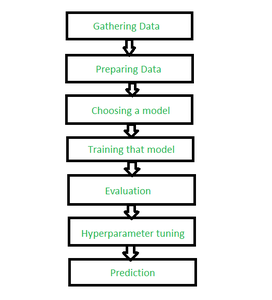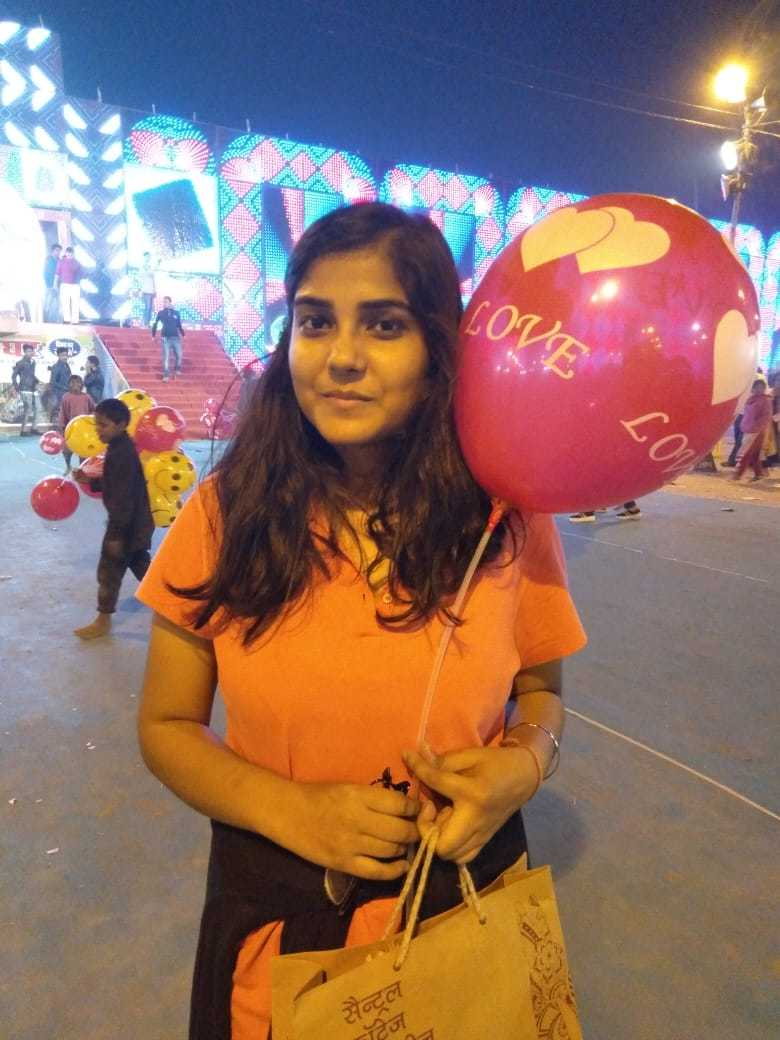# Multi-Label Image Classification – Prediction of image labels

There are so many things we can do using computer vision algorithms:

• Object detection
• Image segmentation
• Image translation
• Object tracking (in real-time), and a whole lot more.

What is Multi-Label Image Classification?
Let’s understand the concept of multi-label image classification with an intuitive example. If I show you an image of a ball, you’ll easily classify it as a ball in your mind. The next image I show you are of a terrace. Now we can divide the two images in two classes i.e. ball or no-ball.
When we have only two classes in which the images can be classified, this is known as a binary image classification problem.

• When there are more than two categories in which the images can be classified.
• An image does not belong to more than one category

If both of the above conditions are satisfied, it is referred to as a multi-class image classification problem.

Prerequisites:

Here, we will be using the following languages and editors:

• Language/Interpreter : Python 3 (preferably python 3.8) from python.org
• Editer : Jupyter iPython Notebook
• OS :  Windows 10 x64
• Python Requirements :This project requires the following libraries to be installed via pip: Numpy, Pandas, MatPlotLib, Scikit Learn, Scikit Image.

In the CMD window, run the following command to install the requirements:

—————————————————————————-

|  pip install numpy pandas matplotlib notebook scikit-image scikit-learn  |

—————————————————————————-

Note :
replace pip with conda if you use anaconda!!

Now run jupyter and open the notebook in the files you downloaded earlier.

Steps to be followed:Steps for label classification

Step 1: Importing the libraries we need.

 `# system libraries ` `import` `os ` `import` `warnings ` ` `  `# ignoring all the warnings ` `warnings.simplefilter(``'ignore'``) ` ` `  `# import data handling libraries ` `import` `numpy as np ` `import` `pandas as pd ` ` `  `# importing data visualisation libraires ` `import` `matplotlib.pyplot as plt  ` `%` `matplotlib inline ` ` `  `# import image processing library ` `from` `skimage.io ``import` `imread, imshow ` `from` `skimage.transform ``import` `resize ` `from` `skimage.color ``import` `rgb2grey`

Step 2: Reading of target images into the project
In this portion of the article, we will be instructing python to read images one by one and then insert the pixel data of the images into arrays that we can use. Then we’ll be creating file lists by Python’s os library.

os.listdir(path) returns a list containing the names of the entries in the directory given by path.

 `r ``=` `os.listdir(r``"C:\Users\Garima Singh\Desktop\data\mugshots\r"``) ` `# This is the path to the image folder ` ` `  `v ``=` `os.listdir(r``"C:\Users\Garima Singh\Desktop\data\mugshots\v"``) ` `d ``=` `os.listdir(r``"C:\Users\Garima Singh\Desktop\data\mugshots\d"``) ` ` `  `print``(r[``0``:``10``])`

Step 3: Creating and importing data from the images and Setting up a limit.
Here, we will use NumPy and scikit-image’s `imread` function. Since we have the downloaded data, we can quickly count how many images per subject we have. For example, suppose you have 100 images in each folder (r, v and d), you can set a variable limit with values 100.  Next step is to create empty arrays for this data and filling up these arrays with data. We will quickly make 3 arrays to accommodate the data of the series of images. We create an array filled with “None” values using the following code snippet:

 `limit ``=` `100`  `# Creating the list of blank spaces that can potentially hold data: ` `ra_images ``=` `[``None``]``*``limit ` ` `  `# Creating loop variables: ` `i, j ``=` `0``, ``0`  ` `  `# This part of the code loops over all the images ` `# in the list "r" and reads it into the jth element  ` `# of the array we made in line 2. ` `for` `i ``in` `r: ` `    ``if``(j

Step 4: Assembly of Data set and Flattening and reshaping of the arrays.
In this section, we will be using pandas Data Frame to merge these 3 data arrays into a single data array. Right now our image array is of size 28×28. We need to make this array into an array of 28^2 x 1. This basically means we have to make take each image and convert it into a row of data in our dataset.

 `# Finding out the number of columns per image in our dataset. ` `# We will use the shape function on any one image in our array ` `# and use the dimensions we get as the number of columns in row. ` `number_of_columns ``=` `ra_grey[``1``].shape[``0``] ``*` `ra_grey[``1``].shape[``1``] ` `print``(number_of_columns) ` ` `  `# This means we will be using this many columns ` `# per row in our dataset. ` `# Our dataset has 300 images, so: ` `# Our dataset will be an array of dimensions  ` `# 784 x 300 => 300 images of 784 pixels each.  `

Step 5: Flattening and Reshaping the data.
This is the part of the code that first converts the 28×28 array into a column vector (i.e. 784 x 1 matrix).

 `print``(ra_grey[``0``].shape) ` `for` `i ``in` `range``(limit): ` `    ``ra_grey[i] ``=` `np.ndarray.flatten(ra_grey[i]).reshape(number_of_columns, ``1``) ` `print``(ra_grey[``0``].shape) ` ` `  `# We will use NumPy's dstack and rollaxis to remove the extra axis(the 1 part in last output) that we saw in the above code output. ` ` `  `ra_grey ``=` `np.dstack(ra_grey) ` `print``(ra_grey.shape) ` `ra_grey ``=` `np.rollaxis(ra_grey, axis ``=` `2``, start ``=` `0``) ` `print``(ra_grey.shape) ` `ra_grey ``=` `ra_grey.reshape(limit, number_of_columns) ` `print``(ra_grey.shape)`

Doing the above for the rest of the data i.e. do the above 2 steps for the next 2 arrays.

Step 6: Converting arrays to dataframes
As discussed before, pandas makes a spreadsheet software like environment for our tables. Lets convert our arrays to dataframes:

 `ra_data ``=` `pd.DataFrame(ra_grey) ` `dh_data ``=` `pd.DataFrame(dh_grey) ` `vi_data ``=` `pd.DataFrame(vi_grey) ` ` `  `ra_data ` ` `  `print``(ra_data)`

Step 7: Adding a name to the images.
In this step we add a column containing the name of our subjects.

This is called labelling our images. The model will try to predict based on the values and it will output one of these labels.

 `ra_data[``"label"``]``=``"R"` `dh_data[``"label"``]``=``"D"` `vi_data[``"label"``]``=``"V"` ` `  `vi_data ` ` `  `# Joining and mixing the data into one dataframe. ` `# First, we will start with joining all 3 dataframes  ` `# made in 3.2 into a single dataframe, using concat function. ` `# Note: It is recommended to join the first 2, ` `# then join the last one into the first pair. ` ` `  `act ``=` `pd.concat([ra_data, dh_data]) ` `actor ``=` `pd.concat([act, vi_data]) ` ` `  `actor`

Step 8: Shuffling the data and Printing the final data set
This is the last stage of this section. We will be shuffling the data, so that it may seem mixed.

 `from` `sklearn.utils ``import` `shuffle ` `out ``=` `shuffle(actor).reset_index() ` ` `  `out ` ` `  `# Drop the column named index ` `out ``=` `out.drop([``'index'``], axis ``=` `1``) ` `out`

Step 9: Coding the machine learning algorithm + Testing Accuracy.
In this section we will code in the machine learning algorithm and find out our algorithm’s accuracy.

 `# First, we will extract the x and y values of our dataset ` ` `  `x ``=` `out.values[:, :``-``1``] ` `y ``=` `out.values[:, ``-``1``] ` ` `  `print``(x[``0``:``3``]) ` `print``(y[``0``:``3``]) ` ` `  `# From the above output, we can see that: ` `# x - stores the image data. ` `# y - stores the label data.`

Step 10: Importing ML libraries and ML Coding
We will import a few ML libraries, all of these will come from sklearn and its classes.

 `from` `sklearn.decomposition ``import` `PCA                    ` `from` `sklearn.svm ``import` `SVC                              ` `from` `sklearn.pipeline ``import` `make_pipeline               ` `from` `sklearn.model_selection ``import` `train_test_split     ` `from` `sklearn.model_selection ``import` `GridSearchCV         ` `from` `sklearn ``import` `metrics                            ` ` `  `# Here we will use train_test_split to create our training and testing data. ` `x_train, x_test, y_train, y_test ``=` `train_test_split(x, y, random_state ``=` `0``) ` ` `  `pca ``=` `PCA(n_components ``=` `150``, whiten ``=` `True``, random_state ``=` `0``) ` `svc ``=` `SVC(kernel ``=``'rbf'``, class_weight ``=``'balanced'``) ` `model ``=` `make_pipeline(pca, svc) ` ` `  `params ``=` `{``'svc__C'``: [x ``for` `x ``in` `range``(``1``, ``6``)], ` `          ``'svc__gamma'``: [``0.001``, ``0.005``, ``0.006``, ``0.01``, ``0.05``, ``0.06``, ``0.004``, ``0.04``]} ` ` `  `grid ``=` `GridSearchCV(model, params) ` `%` `time grid.fit(x_train, y_train) ` `print``(grid.best_params_) ` ` `  `model ``=` `grid.best_estimator_ ` `ypred ``=` `model.predict(x_test) ` ` `  `ypred[``0``:``3``]`

We will use the PCA class and SVC class to create our model object. The make_pipeline will help us create an easy model that can be tested by GridSearchSV.

GridSearchSV is the function that will create a model with all of the parameters in EVERY combination possible and tell us which is the best combination.

Now that we have the model with the best parameters for our data, we use these parameters in our model and test its accuracy.
Step 11: Diagrams and getting accuracy
Lets see a visualized diagram of faces vs predicted labels:

 `fig, ax ``=` `plt.subplots(``4``, ``4``, sharex ``=` `True``,  ` `                             ``sharey ``=` `True``,  ` `                        ``figsize ``=` `(``10``, ``10``)) ` ` `  `for` `i, axi ``in` `enumerate``(ax.flat): ` `    ``axi.imshow(x_test[i].reshape(imsize).astype(np.float64), ` `                   ``cmap ``=` `"gray"``, interpolation ``=` `"nearest"``) ` ` `  `    ``axi.set_title(``'Label : {}'``.``format``(ypred[i])) ` `     `  `# Finally, we test our accuracy in using the following code: ` `print``(metrics.accuracy_score(y_test, ypred) ``*` `100``)`

Conclusion:
Labeling the images to create the training data for machine learning or AI is not a difficult task. You just need the right techniques for it. This articles showed an image labeling process from scratch to mastery.

My Personal Notes arrow_drop_upCheck out this Author's contributed articles.

If you like GeeksforGeeks and would like to contribute, you can also write an article using contribute.geeksforgeeks.org or mail your article to contribute@geeksforgeeks.org. See your article appearing on the GeeksforGeeks main page and help other Geeks.

Please Improve this article if you find anything incorrect by clicking on the "Improve Article" button below.

Article Tags :
Practice Tags :

Be the First to upvote.

Please write to us at contribute@geeksforgeeks.org to report any issue with the above content.